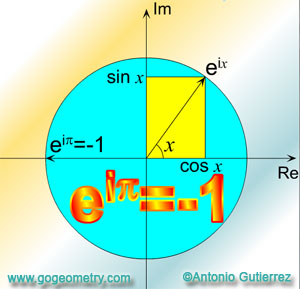# Euler's Formula / Euler's Equation or Identity

 Leonhard Euler (Swiss mathematician and physicist, 1707-1783) and his beautiful and extraordinary formula that links the 5 fundamental constants in Mathematics, namely, e, the base of the natural logarithms, i, the square root of -1, Pi, the ratio of the circumference of a circle to its diameter, 1 and 0, together! Euler's Equation or IdentityEuler's formula is a mathematical formula in complex analysis that shows a deep relationship between the trigonometric functions and the complex exponential function. Euler's formula states that, for any real number x: (1) $$e^{ix}=\cos x+i\cdot \sin x$$  (Euler's formula) where: e is the base of the natural logarithm i is the imaginary unit sin and cos are trigonometric functions. Euler's equation or identity is a special case of the Euler' formula, where: (2) $$x=\pi$$ By substitution in (1): (3) $$e^{i\pi }=\cos \pi +i\cdot \sin \pi$$ (4) $$e^{i\pi }=-1+0$$ (5) Therefore: $$e^{i\pi }=-1$$ (Euler's Equation or Identity)Benjamin Peirce (1809-1880, American mathematician, professor at Harvard) gave a lecture proving "Euler's equation", and concluded: "Gentlemen, that is surely true,it is absolutely paradoxical;we cannot understand it,and we don't know what it means.But we have proved it,and therefore we know it must be the truth."   Reference: The Changing Shape of Geometry. Celebrating a Century of Geometry and Geometry Teaching. Edited on behalf of The Mathematical Association UK by Chris Pritchard. Cambridge University Press, (Cambridge 2003).
 Home | Geometry | Problems | Search | HTML5 Animation for iPad and Nexus | Email | by Antonio Gutierrez